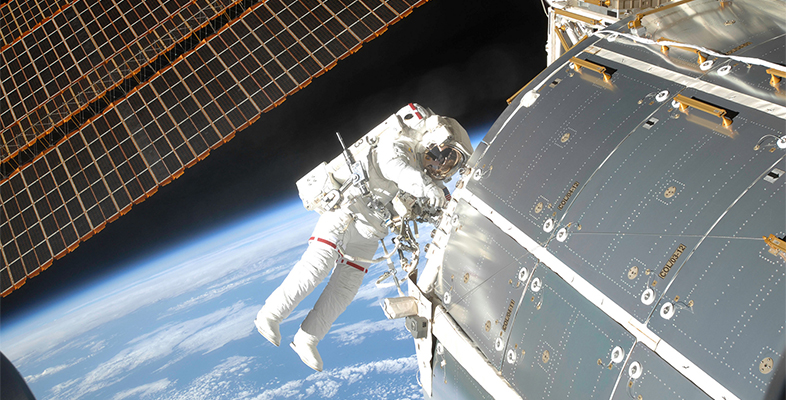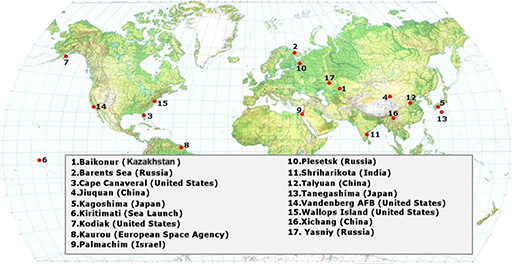Science, Maths & Technology

### Become an OU studentMicrogravity: living on the International Space Station

Start this free course now. Just create an account and sign in. Enrol and complete the course for a free statement of participation or digital badge if available.

# 3.2 Location of launch sites

You should now appreciate how difficult it is to send a rocket into space. But what about the location of the launch site on Earth? Take a look at Figure 11 and then complete Activity 5.Figure 11 Worldwide rocket launch sites.

## Activity 5 Launching a rocket

Timing: Allow approximately 15 minutes

Using the information in Figure 11, answer the following questions.

1. Where do you think the best places on Earth to launch a rocket from are? Choose the one correct option below.

a.

Equator

b.

North Pole

c.

Atlantic Ocean

d.

Pacific Ocean

e.

South Pole

### Discussion

At the Equator, Earth is rotating at nearly 1700 kilometres per hour (km/h). If the rocket is launched half-way between the Equator and the North or South Pole, the speed is reduced by nearly 500 km/h. This makes it harder for the rocket to escape Earth’s gravity.

Now choose the correct options to complete the following statements.

2. The largest number of launch sites are in

a.

Russia and the USA

b.

India and China

c.

Japan and the European Space Agency

The largest number of launch sites are in Russia and the USA.

Active content not displayed. This content requires JavaScript to be enabled.
Interactive feature not available in single page view (see it in standard view).
Active content not displayed. This content requires JavaScript to be enabled.
Interactive feature not available in single page view (see it in standard view).
Active content not displayed. This content requires JavaScript to be enabled.
Interactive feature not available in single page view (see it in standard view).

How can you calculate the acceleration of a rocket from the launch pad to the edge of the Earth’s atmosphere? To do this, you need to use one of Newton’s Laws. Here, you need the Second Law which relates force (F), mass (m) and acceleration (a) as shown in Equation 1.

Equation label: Equation 1

You need to change the mass of the rocket – in this case, say 40 tonnes – into kg. So, the mass m = 40 × 1000 kg = 40 000 kg = 4 × 104 kg.

Question 4 in Activity 5 provided an approximate value of the thrust of such a rocket, that is, F = 800 000 N = 8 × 105 N.

Now, if you rearrange Equation 1 and substitute in these values, you get Equation 2.

Equation label: Equation 2

Here you can see that the derived units of newtons (N) have been broken down into the fundamental units of kilograms (kg), m and s. The numerical value in Equation 2 is just over twice the value of the acceleration due to gravity (g) near the surface of the Earth, which is 9.81 m/s2 (or m s–2). It means that, for every second you fall under Earth’s gravity, you travel another 9.81 m/s faster.

Now you’ve calculated how to get to the ISS. But how does it stay up in Earth’s orbit?